Home

# ADJOINT FUNCTORS BETWEEN CATEGORIES OF HILBERT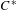$C^{\ast }$ -MODULES

## Abstract

Let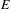$E$ be a (right) Hilbert module over a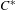$C^{\ast }$ -algebra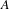$A$ . If$E$ is equipped with a left action of a second$C^{\ast }$ -algebra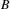$B$ , then tensor product with$E$ gives rise to a functor from the category of Hilbert$B$ -modules to the category of Hilbert$A$ -modules. The purpose of this paper is to study adjunctions between functors of this sort. We shall introduce a new kind of adjunction relation, called a local adjunction, that is weaker than the standard concept from category theory. We shall give several examples, the most important of which is the functor of parabolic induction in the tempered representation theory of real reductive groups. Each local adjunction gives rise to an ordinary adjunction of functors between categories of Hilbert space representations. In this way we shall show that the parabolic induction functor has a simultaneous left and right adjoint, namely the parabolic restriction functor constructed in Clare et al. [Parabolic induction and restriction via$C^{\ast }$ -algebras and Hilbert$C^{\ast }$ -modules, Compos. Math. FirstView (2016), 1–33, 2].

## References

Hide All
MathJax
MathJax is a JavaScript display engine for mathematics. For more information see http://www.mathjax.org.

# ADJOINT FUNCTORS BETWEEN CATEGORIES OF HILBERT$C^{\ast }$ -MODULES

## Metrics

### Full text viewsFull text views reflects the number of PDF downloads, PDFs sent to Google Drive, Dropbox and Kindle and HTML full text views.

Total number of HTML views: 0
Total number of PDF views: 0 *Loading metrics...

### Abstract viewsAbstract views reflect the number of visits to the article landing page.

Total abstract views: 0 *Loading metrics...

* Views captured on Cambridge Core between <date>. This data will be updated every 24 hours.

Usage data cannot currently be displayed